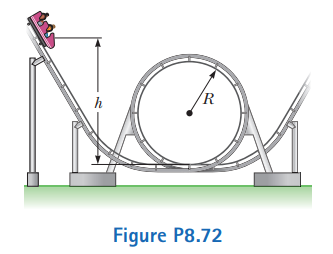# Problem: A roller-coaster car shown in Figure P8.72 is released from rest from a height h and then moves freely with negligible friction. The roller-coaster track includes a circular loop of radius R in a vertical plane.(a) First suppose the car barely makes it around the loop; at the top of the loop, the riders are upside down and feel weightless. Find the required height h of the release point above the bottom of the loop in terms of R.(b) Now assume the release point is at or above the minimum required height. Show that the normal force on the car at the bottom of the loop exceeds the normal force at the top of the loop by six times the car’s weight. The normal force on each rider follows the same rule. Such a large normal force is dangerous and very uncomfortable for the riders. Roller coasters are therefore not built with circular loops in vertical planes. Figure P6.17 (page 170) shows an actual design.

###### FREE Expert Solution

In this problem, we'll be dealing with gravitational potential energy, kinetic energy, and centripetal force/acceleration.

Centripetal acceleration:

$\overline{){\mathbf{a}}{\mathbf{=}}\frac{{\mathbf{v}}^{\mathbf{2}}}{\mathbf{r}}}$

93% (404 ratings)###### Problem Details

A roller-coaster car shown in Figure P8.72 is released from rest from a height h and then moves freely with negligible friction. The roller-coaster track includes a circular loop of radius R in a vertical plane.

(a) First suppose the car barely makes it around the loop; at the top of the loop, the riders are upside down and feel weightless. Find the required height h of the release point above the bottom of the loop in terms of R.

(b) Now assume the release point is at or above the minimum required height. Show that the normal force on the car at the bottom of the loop exceeds the normal force at the top of the loop by six times the car’s weight. The normal force on each rider follows the same rule. Such a large normal force is dangerous and very uncomfortable for the riders. Roller coasters are therefore not built with circular loops in vertical planes. Figure P6.17 (page 170) shows an actual design.Frequently Asked Questions

What scientific concept do you need to know in order to solve this problem?

Our tutors have indicated that to solve this problem you will need to apply the Motion Along Curved Paths concept. You can view video lessons to learn Motion Along Curved Paths. Or if you need more Motion Along Curved Paths practice, you can also practice Motion Along Curved Paths practice problems.

What professor is this problem relevant for?

Based on our data, we think this problem is relevant for Professor Khondaker's class at UCF.

What textbook is this problem found in?

Our data indicates that this problem or a close variation was asked in Physics for Scientists and Engineers - Serway Calc 9th Edition. You can also practice Physics for Scientists and Engineers - Serway Calc 9th Edition practice problems.